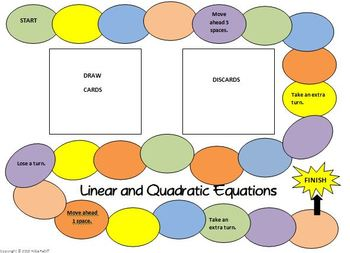DID YOU KNOW:
Seamlessly assign resources as digital activities

Learn how in 5 minutes with a tutorial resource. Try it Now

Learn More# High School Algebra Board Game - Linear and Quadratic Equations9th - 10th
Subjects
Standards
Resource Type
Formats Included
• PDF
Pages
20 pages

### Description

Would you like a different way for your algebra students to practice or review solving linear and quadratic equations? This board game is fun for the students while they sharpen their skills and deepen their understanding of these important concepts. They will draw a card and they must correctly answer the question in order to move along the game board. One person will be designated as the Answer Checker. The rules of the game are explained in the Preview.

The questions on the game cards have students solve equations for a particular variable. Some of the linear equations have variable coefficients. Both linear and quadratic equations may contain rational numbers and grouping symbols.
Students must recognize the quadratic equations and decide what method to use to solve them. Some questions require them to select an equation that has no real solutions.

This product includes the following.
1. Game Board
2. 40 Game Cards
3. Game Pieces

The 40 game cards will allow this game to be used for multiple class periods.

MATH DOMINOES - Solve Linear Equations

MATH DOMINOES - Solve Quadratic Equations

Thanks for viewing!

Created by Hilda Ratliff
Total Pages
20 pages
Included
Teaching Duration
N/A
Report this Resource to TpT
Reported resources will be reviewed by our team. Report this resource to let us know if this resource violates TpT’s content guidelines.

### Standards

to see state-specific standards (only available in the US).
Solve quadratic equations by inspection (e.g., for 𝘹² = 49), taking square roots, completing the square, the quadratic formula and factoring, as appropriate to the initial form of the equation. Recognize when the quadratic formula gives complex solutions and write them as 𝘢 ± 𝘣𝘪 for real numbers 𝘢 and 𝘣.
Solve linear equations and inequalities in one variable, including equations with coefficients represented by letters.